# For the following reaction KClO3---->KCl+3/2 O2 assign oxidation states to each element on each side

For the following reaction KClO3---->KCl+3/2 O2 assign oxidation states to each element on each side of the equation…reactants and products. Which element is oxidized? Which element is reduced?

Concepts and reason
In the given question, a reaction is given. The oxidation state of elements has to be found out either by looking into its group number the periodic table or by calculating it through summing up the oxidation states of all the elements in that compound and equating it to the charge present on it.

Fundamentals
Oxidation: It means that if an element loses an electron, or gains oxygen during a reaction then it is said to be oxidation and that element is said to be oxidized.
Reduction: It means that if an element gains an electron, or loses oxygen during a reaction then it is said to be reduction and that element is said to be reduced.

The given reaction is: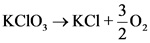The oxidation states of elements of the reactant -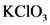are:
Oxidation state of K is +1.
Oxidation state of O is -2.
Calculate oxidation state of Cl as: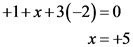The oxidation states of elements of the product -KCI are:
Oxidation state of K is +1.
Calculate oxidation state of Cl as:The oxidation state of O in the product -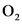is 0.

Oxidation state of elements on each side of the equation are as follows: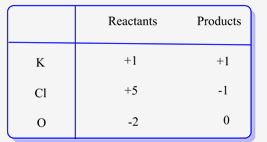For ,since potassium belongs to group IA in the periodic table, its oxidation state is +1. Moreover, oxygen belongs to group VIA, hence, its oxidation state is -2.
Therefore, the oxidation state for Cl is calculated by adding the oxidation states of K and O and equating it to the charge onwhich is neutral.
Similarly, the oxidation state of Cl in KCI is also calculated.

According to the oxidation states calculated in Step 1, oxidation state of oxygen changes from -2 to 0 from reactants to products.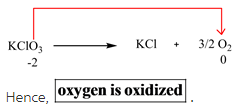The element which is oxidized is oxygen.

Oxidation means that the oxidation state on that element increases when it goes from reactant to product side. Since the oxidation state of oxygen increases from -2 to 0, hence it is oxidized.

According to the oxidation states calculated in Step 1, oxidation state of chlorine changes from +5 to -1 from reactants to products.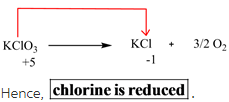The element which is reduced is chlorine.
Reduction means that the oxidation state on that element decreases when it goes from reactant to product side. Since the oxidation state of chlorine decreases from +5 to -1, hence it is reduced.